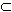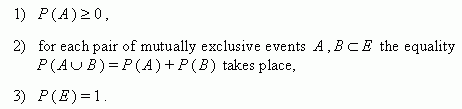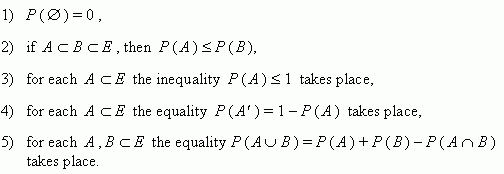Definition and basic properties of probability

Axiomatic and classical definitions of probability.

Probability of event. Basic properties of probability.

The axiomatic definition of probability. Let a space of elementary events E be given and such single number Ð ( À ) corresponds to each event ÀÅ , that:Then we say, that the probability is defined on events of E , and the number Ð ( À ) is called the probability of an event A .

The classical definition of probability . Let a space of elementary events E be given and this space consists of N equally likely elementary events, among which there are n events, favorable for an event A . Then the number

Ð ( À ) = n / N

is called the probability of an event A .

Basic properties of probability . Let a space of elementary events E be given and probabilities P are defined on events of E . Then: